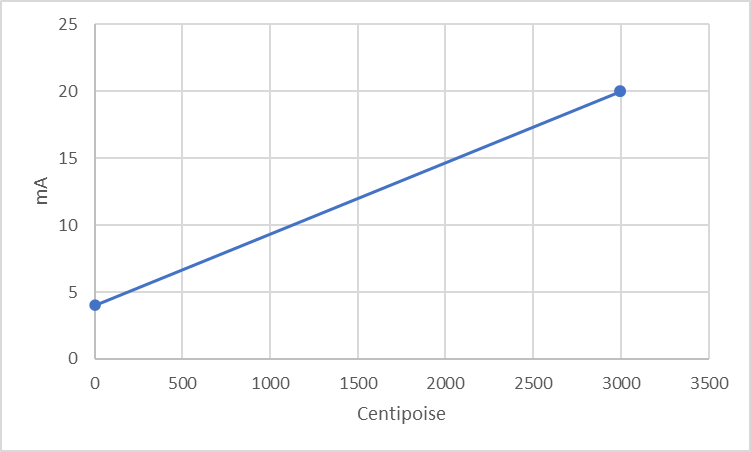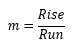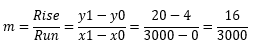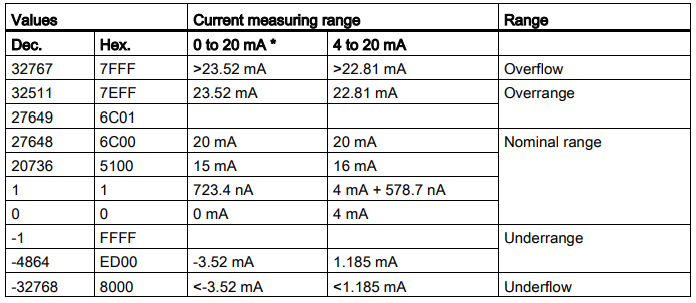A current loop can be modeled with a commonly used equation of a straight line with the form y = mx + b. 4-20mA signals represents a value in 0 - 100% scale.

For Rheonics standard range of viscosity(0-3000cP) this line can be modeled as follows:Where:

y: Instrument Output

x: Instrument Input

m: Slope

b: y-intercept

Finding m and b:In our scenario m is calculated as.b is calculated as the value that intercepts with the y-axis. b is equal to 4.

Using this linear equation, we can predict any value for y given x, and vice versa. To find the correct limits to use with the Rheonics sensor, for example with a viscosity of 1cP for water the signal you get on the analog channel is 4 mA + 1/3000*16 mA = 4.0053 mA ( you get a 0.0053 mA signal for water) so you cannot measure it with anything but an extremely accurate ammeter!

If the range is reduced to 0-10cP, for example, 1cP will give you 4mA + 1/10*16mA = 5.6mA - something that a PLC can actually measure!

`PLC resolution`

For a Siemens card 6ES7134-6GD01-0BA1 with a resolution of 16 bits with a range of -32768 to 32,767 ADC counts, the raw value from the PLC must be scaled to real-life measurements. In our scenario, the low limit will correspond to (4mA-0-0cP) and High Limit (20mA-27648-3000cP)References:

Analog signal Integration with Micro820